# Statistics

## Producer Field Guide

HGD_Product
Producer Field Guide
HGD_Portfolio_Suite
Producer

Histogram

In ERDAS IMAGINE image data files, each data file value (defined by its row, column, and band) is a variable. ERDAS IMAGINE supports the following data types:

• 1, 2, and 4-bit
• 8, 16, and 32-bit signed
• 8, 16, and 32-bit unsigned
• 32 and 64-bit floating point
• 64 and 128-bit complex floating point

Distribution, as used in statistics, is the set of frequencies with which an event occurs, or that a variable has a particular value.

A histogram is a graph of data frequency or distribution. In a histogram, for a single band of data:

• Horizontal axis is the range of all possible data file values
• Vertical axis is the number of pixels that have each data value

Histogram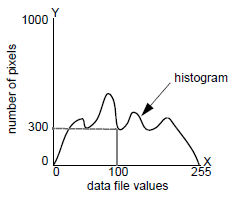This figure shows the histogram for a band of data in which Y pixels have data value X. For example, in this graph, 300 pixels (y) have the data file value of 100 (x).

Bin Functions

Bins are used to group ranges of data values together for better manageability. Histograms and other descriptor columns for 1, 2, 4, and 8-bit data are easy to handle since they contain a maximum of 256 rows. However, to have a row in a descriptor table for every possible data value in floating point, complex, and 32-bit integer data would yield an enormous amount of information. Therefore, the bin function is provided to serve as a data reduction tool.

Example of a Bin Function

Suppose you have a floating point data layer containing values ranging from 0.0 to 1.0. You could set up a descriptor table of 100 rows, where each row or bin corresponds to a data range of 0.01 in the layer.

The bins would look like the following:

 Bin Number Data Range 0 X < 0.01 1 2 ... ... ... 98 99

Then, for example, row 23 of the histogram table would contain the number of pixels in the layer whose value fell between 0.023 and 0.024.

Types of Bin Functions

Bin function establishes the relationship between data values and rows in the descriptor table. There are four types of bin functions used in ERDAS IMAGINE image layers:

• DIRECT—one bin per integer value. Used by default for 1, 2, 4, and 8-bit integer data, but may be used for other data types as well. The direct bin function may include an offset for negative data or data in which the minimum value is greater than zero.

For example, a direct bin with 900 bins and an offset of -601 would look like the following:

 Bin Number Data Range 0 1 ... ... ... 599 600 601 602 603 ... ... ... 898 899
• LINEAR—establishes a linear mapping between data values and bin numbers, as in our first example, mapping the data range 0.0 to 1.0 to bin numbers 0 to 99.

The bin number is computed by:

bin = numbins * (x - min) / (max - min)

if (bin < 0) bin = 0

if (bin >= numbins) bin = numbins - 1

Where:

bin = resulting bin number

numbins = number of bins

x = data value

min = lower limit (usually minimum data value)

max = upper limit (usually maximum data value)

• LOG—establishes a logarithmic mapping between data values and bin numbers. The bin number is computed by:

bin = numbins * (ln (1.0 + ((x - min)/(max - min)))/ ln (2.0))

if (bin < 0) bin = 0

if (bin >= numbins) bin = numbins - 1

• EXPLICIT—explicitly defines mapping between each bin number and data range.

Mean

The mean (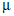) of a set of values is its statistical average, such that, if Qi represents a set of k values: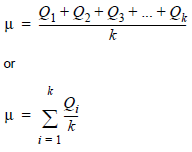The mean of data with a normal distribution is the value at the peak of the curve—the point where the distribution balances.

Normal Distribution

Our general ideas about an average, whether it be average age, average test score, or the average amount of spectral reflectance from oak trees in the spring, are made visible in the graph of a normal distribution, or bell curve.

Normal Distribution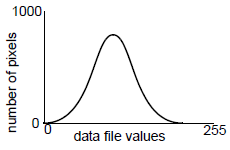Average usually refers to a central value on a bell curve, although all distributions have averages. In a normal distribution, most values are at or near the middle, as shown by the peak of the bell curve. Values that are more extreme are more rare, as shown by the tails at the ends of the curve.

Normal Distributions are a family of bell shaped distributions that turn up frequently under certain special circumstances. For example, a normal distribution would occur if you were to compare the bands in a desert image. The bands would be very similar, but would vary slightly.

Each Normal Distribution uses just two parameters,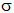and, to control the shape and location of the resulting probability graph through the equation: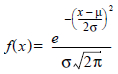Where:

x = quantity’s distribution that is being approximated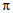and e = famous mathematical constants

The parametercontrols how much the bell is shifted horizontally so that its average matches the average of the distribution of x.

The parameteradjusts the width of the bell to try to encompass the spread of the given distribution.

In choosing to approximate a distribution by the nearest of the Normal Distributions, we describe the many values in the bin function of its distribution with just two parameters. It is a significant simplification that can greatly ease the computational burden of many operations, but like all simplifications, it reduces the accuracy of the conclusions we can draw.

The normal distribution is the most widely encountered model for probability. Many natural phenomena can be predicted or estimated according to the law of averages that is implied by the bell curve (Larsen and Marx, 1981).

A normal distribution in remotely sensed data is meaningful—it is a sign that some characteristic of an object can be measured by the average amount of electromagnetic radiation that the object reflects. This relationship between the data and a physical scene or object is what makes image processing applicable to various types of land analysis.

The mean and standard deviation are often used by computer programs that process and analyze image data.

Variance

The mean of a set of values locates only the average value—it does not adequately describe the set of values by itself. It is helpful to know how much the data varies from its mean. However, a simple average of the differences between each value and the mean equals zero in every case, by definition of the mean. Therefore, the squares of these differences are averaged so that a meaningful number results (Larsen and Marx, 1981).

In theory, the variance is calculated as follows: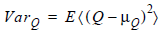Where:

E = expected value (weighted average)

2 = squared to make the distance a positive number

In practice, the use of this equation for variance does not usually reflect the exact nature of the values that are used in the equation. These values are usually only samples of a large data set, and therefore, the mean and variance of the entire data set are estimated, not known.

The equation used in practice follows. This is called the minimum variance unbiased estimator of the variance, or the sample variance (notated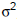).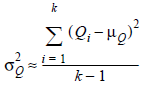Where:

i = a particular pixel

k = number of pixels (the higher the number, the better the approximation)

The theory behind this equation is discussed in chapters on point estimates and sufficient statistics, and covered in most statistics texts.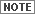Variance is expressed in units squared (for example, square inches, square data values, and so forth), so it may result in a number that is much higher than any of the original values.

Standard Deviation

Since the variance is expressed in units squared, a more useful value is the square root of the variance, which is expressed in units and can be related back to the original values (Larsen and Marx, 1981). The square root of the variance is the standard deviation.

Based on the equation for sample variance (), the sample standard deviation (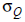) for a set of values Q is computed as follows: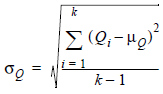In any distribution:

• approximately 68% of the values are within one standard deviation of, that is, between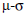and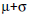• more than 1/2 of the values are between
• more than 3/4 of the values are between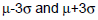An example of a simple application of these rules is seen in ERDAS IMAGINE Viewer. When 8-bit data are displayed in the Viewer, ERDAS IMAGINE can apply a 2 standard deviation stretch that remaps all data file values between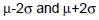(more than 1/2 of the data) to the range of possible brightness values on the display device.

Standard deviations are used because the lowest and highest data file values may be much farther from the mean than 2.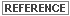For more information on contrast stretch, see Enhancement.

Parameters

As described above, the standard deviation describes how a fixed percentage of the data varies from the mean. The mean and standard deviation are known as parameters, which are sufficient to describe a normal curve (Johnston, 1980).

When the mean and standard deviation are known, they can be used to estimate other calculations about the data. In computer programs, it is much more convenient to estimate calculations with a mean and standard deviation than it is to repeatedly sample the actual data.

Algorithms that use parameters are parametric. The closer that the distribution of the data resembles a normal curve, the more accurate the parametric estimates of the data are. ERDAS IMAGINE classification algorithms that use signature files (.sig) are parametric, since the mean and standard deviation of each sample or cluster are stored in the file to represent the distribution of the values.

Covariance

In many image processing procedures, the relationships between two bands of data are important. Covariance measures the tendencies of data file values in the same pixel, but in different bands, to vary with each other, in relation to the means of their respective bands. These bands must be linear.

Theoretically speaking, whereas variance is the average square of the differences between values and their mean in one band, covariance is the average product of the differences of corresponding values in two different bands from their respective means. Compare the following equation for covariance to the previous one for variance: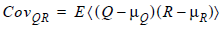Where:

Q and R = data file values in two bands

E = expected value

In practice, the sample covariance is computed with this equation: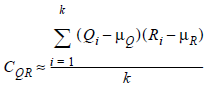Where:

i = a particular pixel

k = number of pixels

Like variance, covariance is expressed in units squared.

Covariance Matrix

Covariance matrix is an n × n matrix that contains all of the variances and covariances within n bands of data. Below is an example of a covariance matrix for four bands of data:

 band A band B band C band D band A VarA CovBA CovCA CovDA band B CovAB VarB CovCB CovDB band C CovAC CovBC VarC CovDC band D CovAD CovBD CovCD VarD

The covariance matrix is symmetrical—for example, CovAB = CovBA.

The covariance of one band of data with itself is the variance of that band: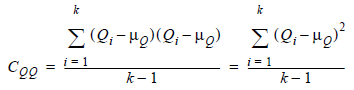Therefore, the diagonal of the covariance matrix consists of the band variances.

The covariance matrix is an organized format for storing variance and covariance information on a computer system, so that it needs to be computed only once. Also, the matrix itself can be used in matrix equations, as in principal components analysis.See Matrix Algebra for more information on matrices.